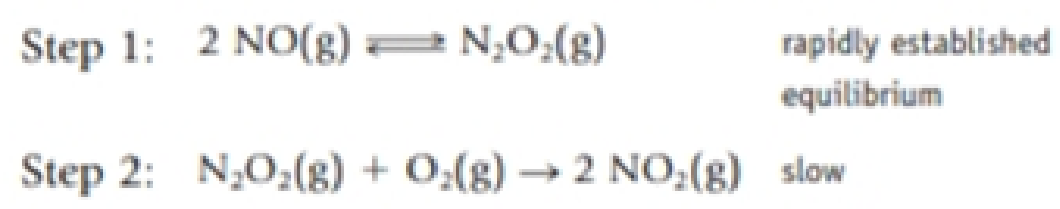Chapter 14, Problem 75GQ

Chapter
Section
Textbook Problem

A reaction that occurs in our atmosphere is the oxidation of NO to the brown gas NO2.2 NO(g) + O2(g) → 2 NO2(g)The mechanism of the reaction is thought to beWhich is the rate determining step? Is there an intermediate in the reaction? If this is the correct mechanism for this reaction, what is the experimentally determined rate law?

Interpretation Introduction

Interpretation:

For the given reaction the rate determining step, intermediate, the rate law should be determined and to state that whether the given mechanism is correct for the reaction.

Concept introduction:

In order to establish the plausibility of a mechanism, one must compare the rate law of the rate determining step to the experimentally determined rate law.

Rate determining step: In a chemical reaction the rate determining step is the slowest step in which the rate of the reaction depends on the rate of that slowest step.

Rate law: It is generally the rate equation that consists of the reaction rate with the concentration or the pressures of the reactants and constant parameters.

Activation energy: It is defined as the minimum energy required by the reacting species in order to undergo chemical reaction.

Rate constant: The rate constant for a chemical reaction is the proportionality term in the chemical reaction rate law which gives the relationship between the rate and the concentration of the reactant present in the chemical reaction.

Intermediate species: It is the species formed during the middle of the chemical reaction between the reactant and the desired product.

Rate order: The order of each reactant in a reaction is represented by the exponential term of the respective reactant present in the rate law and the overall order of the reaction is the sum of all the exponents of all reactants present in the chemical reaction.  The order of the reaction is directly proportional to the concentration of the reactants.

Arrhenius equation:

• Arrhenius equation is a formula that represents the temperature dependence of reaction rates
• The Arrhenius equation has to be represented as follows

k=AeEa/RTlnk=lnAeEa/RTlnk=(EaR)(1T)+lnA

• Ea represents the activation energy and it’s unit is kJ/mol
• R represents the universal gas constant and it has the value of 8.314 J/K.mol
• T represents the absolute temperature
• A represents the frequency factor or collision frequency
• e represents the base of natural logarithm
•  Arrhenius equation equation was proposed by Svante Arrhenius in 1889.
Explanation

Examining the given chemical equations shows that the given reaction is a two-step process in which the first step is fast reversible step whereas the second step is a slow one.

Therefore, the second step that is Step 2 serves as the rate determining step since the slowest step of the reaction serves as the rate determining step since the reaction rate depends on the slowest step.

The species Nitrogen peroxide serves as the intermediate since it is formed between the reactant and the product of the chemical reaction.

The rate determining step for the given chemical reaction is as follows,

N2O2(g)+O2(g)2NO2(g)

The rate law of a chemical reaction is an expression that relates the concentration of the reactant with the rate

Still sussing out bartleby?

Check out a sample textbook solution.

See a sample solution

The Solution to Your Study Problems

Bartleby provides explanations to thousands of textbook problems written by our experts, many with advanced degrees!

Get Started